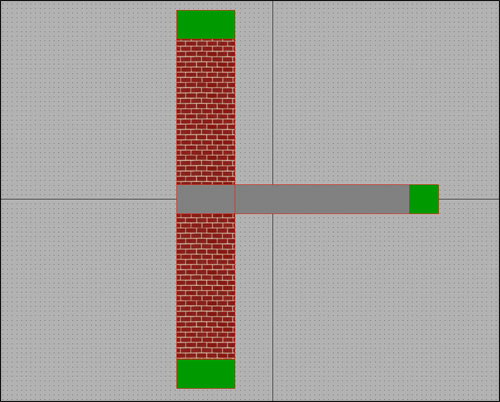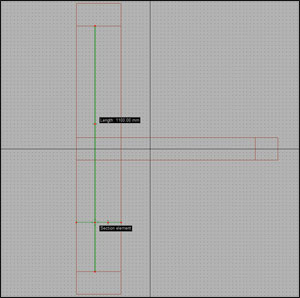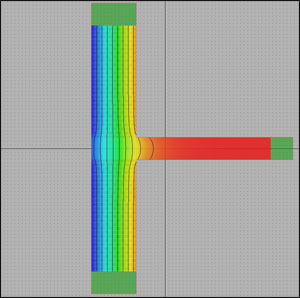# Thermal Bridge Computation

In this brief tutorial we’ll show you how to computer linear thermal transmittance of a typical thermal bridge: a junction between a wall and a floor. We’ll use Mold Simulator as FEM software for its analysis.

## Thermal bridgeThe structure is in contact with external environment (on the left) and internal environment (on the right).
In this case, the thermal bridge is caused by floor; a way to evaluate it is to compute its linear thermal transmittance, ψ (psi) in W/mK.

Linear thermal transmittance
This value represents the difference between the theoretical configuration (just a wall) and the real one (a wall with an intersecting floor).

 Theoretical configuration Real configurationWall length (l): 1.1 m Wall transmittance (U): 1.0980 W/m²K (computed simply considering wall’s material properties) Thermal conductance (L2D): U x l = 1.2078 W/mK Wall length (l): 1.1 m Wall transmittance (U): 1.2548 W/m²K (FEM simulation with Mold Simulator) Thermal conductance (L2D): U x l = 1.3803 W/mK (FEM simulation with Mold Simulator)

Thermal bridge ψ = 1.3803 – 1.2078 = 0.1725 W/mK